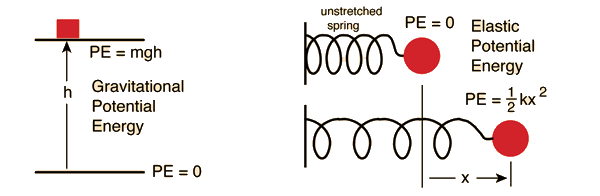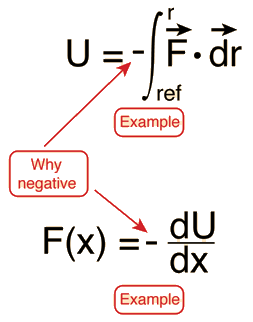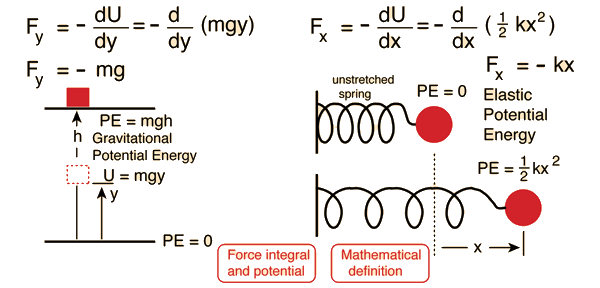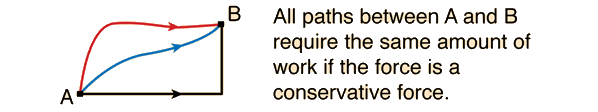Potential Energy

Potential energy is energy which results from position or configuration. The SI unit for energy is the joule = newton x meter in accordance with the basic definition of energy as the capacity for doing work. An object may have the capacity for doing work as a result of its position in a gravitational field (gravitational potential energy), an electric field (electric potential energy), or a magnetic field (magnetic potential energy). It may have elastic potential energy as a result of a stretched spring or other elastic deformation.Index

Mathematical definition

Energy concepts

 HyperPhysics***** Mechanics R Nave
Go Back

Potential Energy Function

If a force acting on an object is a function of position only, it is said to be a conservative force, and it can be represented by a potential energy function which for a one-dimensional case satisfies the derivative conditionThe integral form of this relationship iswhich can be taken as a definition of potential energy. Note that there is an arbitrary constant of integration in that definition, showing that any constant can be added to the potential energy. Practically, this means that you can set the zero of potential energy at any point which is convenient.

Index

Energy concepts

 HyperPhysics***** Mechanics R Nave
Go Back

Potential Energy ConceptThe potential energy U is equal to the work you must do to move an object from the U=0 reference point to the position r. The reference point at which you assign the value U=0 is arbitrary, so may be chosen for convenience, like choosing the origin of a coordinate system. The force on an object is the negative of the derivative of the potential function U. This means it is the negative of the slope of the potential energy curve. Plots of potential functions are valuable aids to visualizing the change of the force in a given region of space.
Index

Energy concepts

 HyperPhysics***** Mechanics R Nave
Go Back

Negative Signs in PotentialF in the definition of potential energy is the force exerted by the force field, e.g., gravity, spring force, etc. The potential energy U is equal to the work you must do against that force to move an object from the U=0 reference point to the position r. The force you must exert to move it must be equal but oppositely directed, and that is the source of the negative sign. The force exerted by the force field always tends toward lower energy and will act to reduce the potential energy. The negative sign on the derivative shows that if the potential U increases with increasing r, the force will tend to move it toward smaller r to decrease the potential energy.
Index

Energy concepts

 HyperPhysics***** Mechanics R Nave
Go Back

Potential Energy Derivative

If the potential energy function U is known, the force at any point can be obtained by taking the derivative of the potential.Index

Energy concepts

 HyperPhysics***** Mechanics R Nave
Go Back

Potential Energy Integral

If the force is known, and is a conservative force, then the potential energy can be obtained by integrating the force.Index

Energy concepts

 HyperPhysics***** Mechanics R Nave
Go Back

Conservative Force

A conservative force may be defined as one for which the work done in moving between two points A and B is independent of the path taken between the two points. The implication of "conservative" in this context is that you could move it from A to B by one path and return to A by another path with no net loss of energy - any closed return path to A takes net zero work.

A further implication is that the energy of an object which is subject only to that conservative force is dependent upon its position and not upon the path by which it reached that position. This makes it possible to define a potential energy function which depends upon position only.Index

Energy concepts

 HyperPhysics***** Mechanics R Nave
Go Back